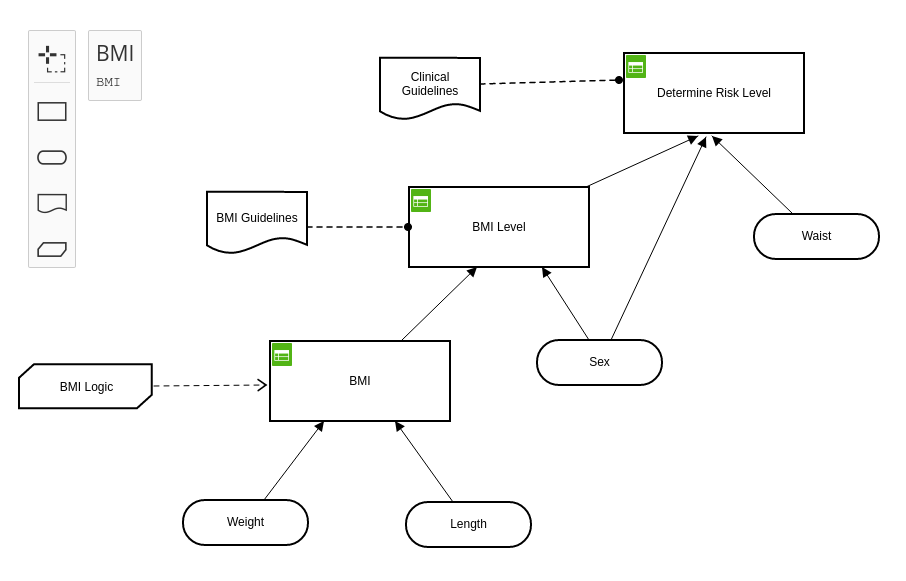Note

We are always happy to support you if you have questions or encounter problems. Feel free to contact us at s.vandevelde@kuleuven.be or by leaving an issue on our issue tracker.

# Python DMN API¶

The cDMN Python package now also comes with a DMN API, that can be used from inside a Python program. It has the following features:

• Query information about the DMN
• Retrieve list of variables
• Retrieve dependencies of variables
• Set the values of certain variables
• Propagate the values throughout the model
• and more

## Start¶

In order to use the DMN API, start by importing the module.

```from cdmn.API import DMN
```

The next step is to load a DMN specification. This specification can either be given as a string (using the `str` argument) or as a path to a `.dmn` file. We will demonstrate this using the BMILevel.dmn example.

```specification = DMN('./path/to/bmi.dmn')
```

If you would like to try out this example for yourself, you may download the DMN file. For context, this is what the example model looks like:BMI
U Weight (kgs) Length (m) BMI
1 - - Weight / (Length * Length)

BMI Level
U BMI Sex BMI Level
1 < 18.5 "Male" "Severely Underweight"
2 < 18.5 "Female" "Underweight"
3 [18.5..24.9] "Male" "Underweight"
4 [18.5..24.9] "Female" "Normal"
5 [25..29.9] "Male" "Normal"
6 [25..29.9] "Female" "Overweight"
7 [30..34.9] - "Obese I"
8 [35..39.9] - "Obese II
9 ≥ 40 - "Extreme Obesity"

BMI
U BMI Level Sex Waist (cm) Risk Level
1 "Overweight" "Male" < 102 "Increased"
2 "Overweight" "Male" ≥ 102 "High"
3 "Overweight" "Female" < 88 "Increased"
4 "Overweight" "Female" ≥ 88 "High"
5 "Obese I" "Male" < 102 "High"
6 "Obese I" "Male" ≥ 102 "Very High"
7 "Obese I" "Female" < 88 "High"
8 "Obese II" "Female" ≥ 88 "Very High"
9 "Extreme Obesity" - - "Very High"
10 "Extreme Obesity" - - "Extremely High"
11 not("Overweight", "Obese I", "Obese II", "Extreme Obesity") - - "Low"

## Query Information¶

Now that our DMN model has been loaded, we can query information such as inputs, outputs and other variables using `get_inputs()`, `get_outputs()` and `get_intermediary()`.

```print("inputs:", spec.get_inputs())
print("outputs:", spec.get_outputs())
print("other:", spec.get_intermediary())
```

results in:

```inputs: ['weight', 'sex', 'length', 'waist']
outputs: ['riskLevel']
other: ['BMILevel', 'bmi']
```

The API also allows us to query dependencies of tables. For example, if we want to find out what variables need to be set in order to calculate the BMI, we can use the `dependencies_of` method. This returns every dependency, together with their “distance” to the variable.

```print('Dependencies of BMI:', spec.dependencies_of('bmi'))
print('Dependencies of riskLevel:\n', spec.dependencies_of('riskLevel')
```

results in:

```Dependencies of BMI: {'weight': 0, 'length': 0}
Dependencies of riskLevel: {'BMILevel': 0, 'bmi': 1, 'weight': 2, 'length': 2, 'sex': 0, 'waist': 0}
```

We can also query the possible values of a type.

```print(spec.possible_values_of('riskLevel'))
```
```High, Increased, Very High, Low, Extremely_High
```

## Interacting with a DMN Model¶

Besides viewing information, we are also able to enter our own data, and actually put the DMN model to use. Setting the value of a variable can be done using the `set_value` method.

```spec.set_value('weight', 74)
spec.set_value('length', 1.79)
```

After setting values, it makes sense to infer information with them. Currently, the DMN API allows for two “inference tasks”. These methods translate the DMN model into an IDP specification, which is then executed.

• `model_expand`: find a set of assignments to the variables that satisfies the DMN specification.
• `propagation`: using the information that we currently have, find out if we can derive the values of other variables.

### Model Expansion¶

Model expansion can be used as such:

```print(spec.model_expand().getvalue())
```

This will print a max of 10 solutions (“models”) in the following form:

```Model 1
==========
BMILevel:={->Underweight}
bmi:={->23.09540900720951}
weight:={->74}
sex:={->Male}
riskLevel:={->Low}
length:={->1.79
```

### Propagation¶

Using the propagation inference, we attempt to infer the value of other variables. In other words: “using our current assignment of variables, are there any other variables that have a known value (e.g. can only have 1 value)?” After executing the propagation, we can use the `is_certain()` method to query whether a variable is certainly known.

For example:

```spec.propagate()
if spec.is_certain('bmi'):
print('BMI:', spec.value_of('bmi'))

if spec.is_certain('riskLevel'):
print('riskLevel:', spec.value_of('riskLevel'))

if spec.is_certain('BMILevel'):
print('BMILevel:', spec.value_of('BMILevel'))
else:
print('BMILevel is still unknown.')
```

results in the following output:

```BMI: 23.09540900720951
riskLevel: Low
BMILevel is still unknown.
```

Because the length and weight were already known, the system was capable of deriving that `BMI` is also known (table 1). Furthermore, the value of `riskLevel` was also derived (table 2). The `BMILevel` on the other hand, can still have multiple values.

#### Autopropagation¶

It is also possible to have the system automatically apply the propagation inference whenever a new variable is entered. When loading in a DMN file, supply the `auto_propagate` flag as follows:

```spec = DMN(path='./path/to/bmi.dmn', auto_propagate=True)

spec.set_value('weight', 74)
spec.set_value('length', 1.79)
if spec.is_certain('bmi'):
```
```Your BMI: 23.095
```

#### Multidirectionality¶

A feature of our DMN API is that it has no sense of directionality. I.e., it is not necessary to use the DMN specification from inputs to outputs. In the following example, we calculate the BMI as before. However, if the BMI is too high, we also calculate what weight the patient should have in order to be healthy. In this way, the propagation can work ‘backwards’, using the same logic!

```spec.clear()  # clears all the previously set values

spec.set_value('weight', 97)
spec.set_value('length', 1.79)

if spec.is_certain('bmi') and float(spec.value_of('bmi')) > 25:
# Get current weight.
cur_weight = spec.value_of('weight')

# Calculate ideal weight.
spec.set_value('weight', None)
spec.set_value('bmi', 25)
ideal_weight = float(spec.value_of('weight'))
print("For a healthy bmi, you should weigh {} kg.".format(ideal_weight))
print("This is a difference of {} kg.".format(round(cur_weight - ideal_weight, 2)))
```
```Your bmi is: 30.273711806747606